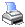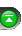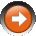﻿ Moving Average - Simple (SMA)
 NinjaScript > Language Reference > Indicator Methods > Moving Average - Simple (SMA)Description

The Simple Moving Average is calculated by summing the closing prices of the security for a period of time and then dividing this total by the number of time periods. Sometimes called an arithmetic moving average, the SMA is basically the average stock price over time.

Syntax

 SMA(int period) SMA(IDataSeries input, int period)   Returns default value SMA(int period)[int barsAgo] SMA(IDataSeries input, int period)[int barsAgo]

Return Value

double; Accessing this method via an index value [int barsAgo] returns the indicator value of the referenced bar.

Parameters

 input Indicator source data (?) period Number of bars used in the calculation

Examples

 // Prints the current value of a 20 period SMA using default price type double value = SMA(20); Print("The current SMA value is " + value.ToString());   // Prints the current value of a 20 period SMA using high price type double value = SMA(High, 20); Print("The current SMA value is " + value.ToString());

Source Code

You can view this indicator method source code by selecting the menu Tools > Edit NinjaScript > Indicator within the NinjaTrader Control Center window.